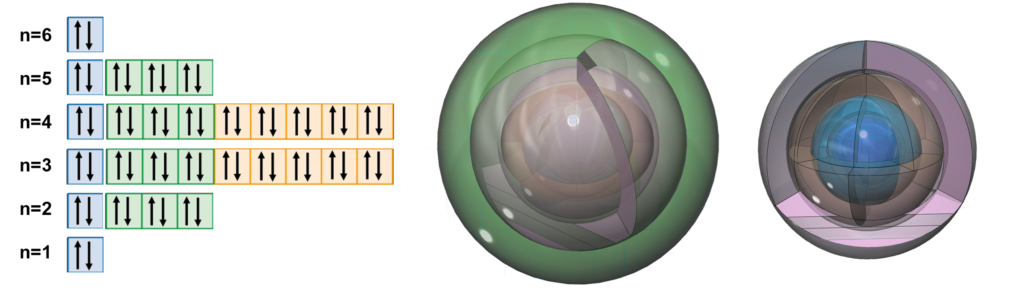# Group II

The elements of Group II are called the Alkali Earth Metals. This is because, when they react with water, they also produce an alkaline (basic) solution. For example, calcium (Ca) reacts with water (H2O) to form calcium hydroxide (Ca(OH)2), which is a strong base. The base of a Group II metal, though, is not as strong as the base from the Group I metal to its left because the ions of Group II are less soluble than the ions of Group I. This means they release fewer hydroxide (OH) ions into solution.

PERIODIC TRENDS:
Effective nuclear charge (Zeff) decreases down the group.
Therefore:
Atomic radius increases down the group.
Ionization energy decreases down the group.
Electronegativity decreases down the group.
Magnetic susceptibility increases down the group.

Since Group II elements have a higher effective nuclear charge (Zeff) than the element preceding them, a Group II element will be slightly less reactive than the Group I element to its left. As we move down the second group, elements become larger, with their valence electrons further from the attraction of the nucleus. The more inner electrons an atom has, the more heavily shielded are the valence electrons from the attraction of the nucleus. This causes each successive element down the group to have lower electronegativity, lower ionization energy, and greater reactivity than the element above it in the group. The base strength of a metal’s hydroxide therefore increases as we go down the group, so strontium hydroxide (Sr(OH)2) is a stronger base than calcium hydroxide (Ca(OH)2), which is a stronger base than magnesium hydroxide (Mg(OH)2). We also see that magnetic susceptibility to an external magnetic field also generally increases as we move down the second group, with the exception of barium’s value (see below).

BERYLLIUM (4Be)
Beryllium has a stable 2s di-electron, and is therefore less reactive than lithium (3Li) and the least reactive of the Group II elements. With only two concentric s-shells, it is also the smallest of the Group II elements (excluding 2He). It therefore has the highest electronegativity (χe=1.57) and the highest ionization energy in the group. Beryllium is diamagnetic (χm=–9), which means that it repels a magnetic field because its outer electrons are strongly aligned with each other, and therefore resist an external magnetic field.

MAGNESIUM (12Mg)
Magnesium is significantly larger than beryllium and its 3s2 di-electron therefore occupies more space than a 2s2 di-electron. The outer electrons are also further from the attraction of the nucleus, giving magnesium a lower electronegativity (χe=1.31) and a lower ionization energy, and making it slightly more reactive than beryllium… though not yet very reactive. Magnesium is paramagnetic (χm=+13.1), which means that it is attracted to a magnetic field because its outer electrons can align individually with an external magnetic field.

CALCIUM (20Ca)
Calcium is larger than magnesium, and also has a full 3rd shell within it. Since there are more electrons shielding the charge of the nucleus, calcium’s outer electrons are much less strongly bound than in magnesium, making calcium much more reactive than magnesium with a lower electronegativity (χe=1.00) and a much lower ionization energy. Since calcium’s outer electrons are less well-bound to one another, calcium is also more strongly paramagnetic (with χm=+40) than magnesium.

STRONTIUM (38Sr)
Strontium is larger than calcium, and also has a full 4th shell within it, now featuring a full d-orbital as well. Since there are more electrons shielding the charge of the nucleus, strontium’s outer electrons are less strongly bound than in calcium, making it much more reactive than calcium with a lower electronegativity (χe=0.95) and a lower ionization energy. The less well-bound outer electrons of strontium make it more strongly paramagnetic (with χm=+92) than calcium.

BARIUM (56Ba)
Barium is larger than strontium, and therefore has a lower electronegativity (χe=0.89) and a lower ionization energy, rendering it more reactive than strontium. It also has a full 5th shell within it, now featuring two full d-orbitals and a remarkable electron orbital symmetry (see below). While there are again more electrons shielding the charge of the nucleus, and barium’s outer electrons would seem less strongly bound than in strontium, barium is nevertheless significantly less paramagnetic (with χm=+20) than strontium, and even than calcium. This would either mean that the outer 6s2 di-electron is more well-bound, as a di-electron, than are the di-electrons of the 5s2 and 4s2 orbitals, which seems unlikely, or else it suggests that the electron symmetry of barium, depicted below, may result in a more coherent harmonic electron state than the elements above it on the periodic table can achieve.The highly symmetrical electron configuration of barium (left), barium’s 6 shells (middle), and with orbitals removed (right) for ease of viewing.

RADIUM (88Ra) is not shown here.

PERIODIC TRENDS: PERIOD II, PERIOD III, GROUP I, GROUP II, GROUP VII, GROUP VIII